Transcripted Summary

All the tests that we created up to this point did not do anything other than just do `System.out` to the console.

In reality, in our tests we need to check for things, whether we are doing API tests or we are doing UI tests.

For any action that we perform, we might need to perform some checking.

We might need to check that certain values equal other values, we might check that some boolean conditions are true or false, or maybe some other conditions.

In order to perform these checks, we will use Assertions, which are basically, let's say, one of the main features of the JUnit 5 framework.

In this chapter, we will go over the Assertions that are JUnit specific.

Let's create a new class where we will have all our assertion related tests.

I will call this new class the `AssertionsTest` class.

We will create a few test methods.

Each of them will demonstrate a different assertion.

I will create a first one, which will say `assertEqualsTest`.

We can start with `Assertions.`, which is the class from the `junit.jupiter.api` package, where we will find all of the assertions.

Then we will have all of the options available in the IDE, and we can choose whatever assertion method we want from this class.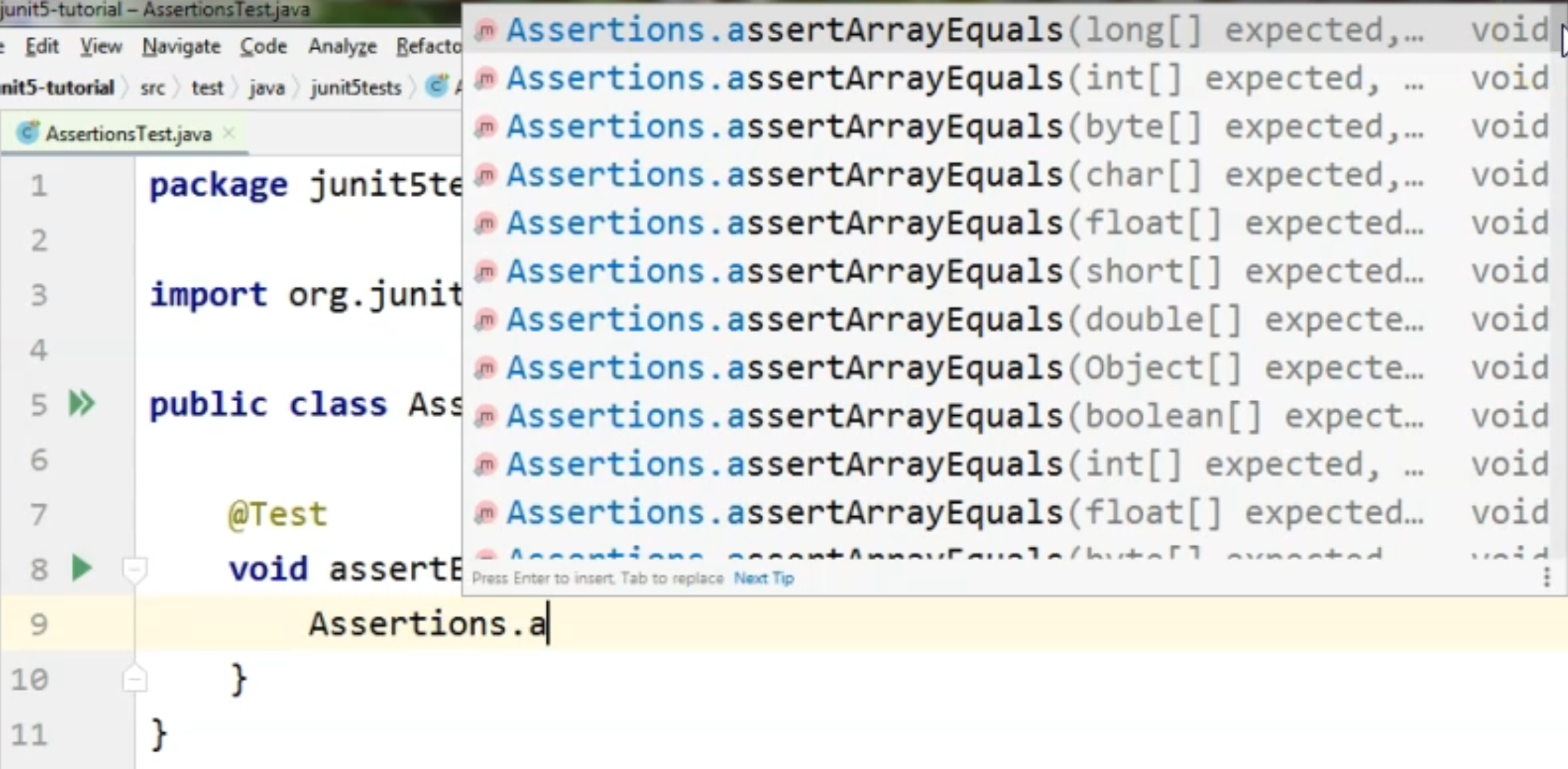As I said here, we will deal with the `assertEquals` so we'll just say, `Assertions.assertEquals()`.

As you have seen, we have quite a few options available.

I will do an "Alt+Enter" here so that I import the Assertions class and I will go back to my method, `assertEquals()`.

As you can see in the overlay, we have the option to compare parameters of different types.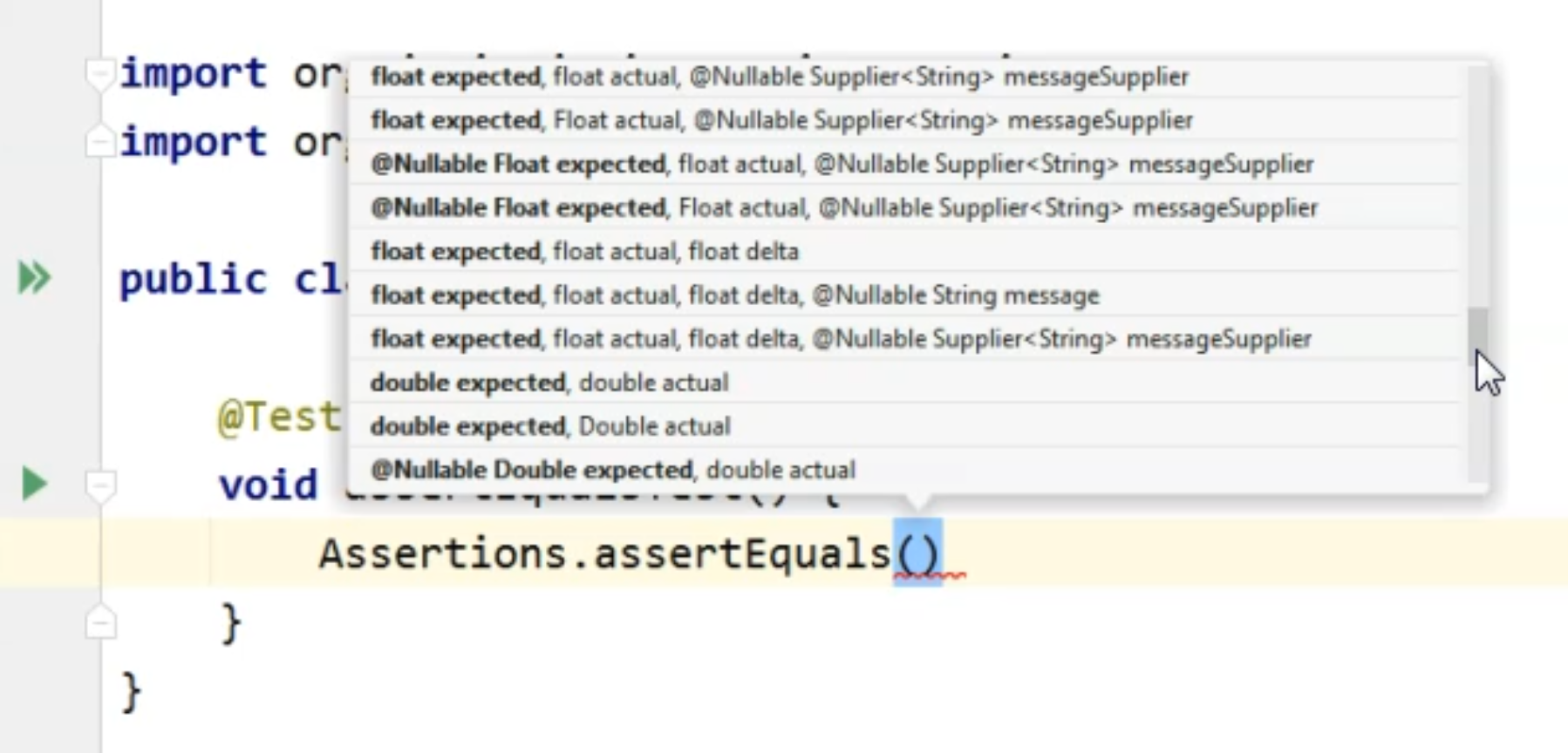We can compare strings, ints, floats, doubles and so on.

We will start with a very simple example where we will just compare two strings.

I will just say `assertEquals("firstString", "secondString")`.

In our case, of course, because the two strings are not equal, this Assertion will fail.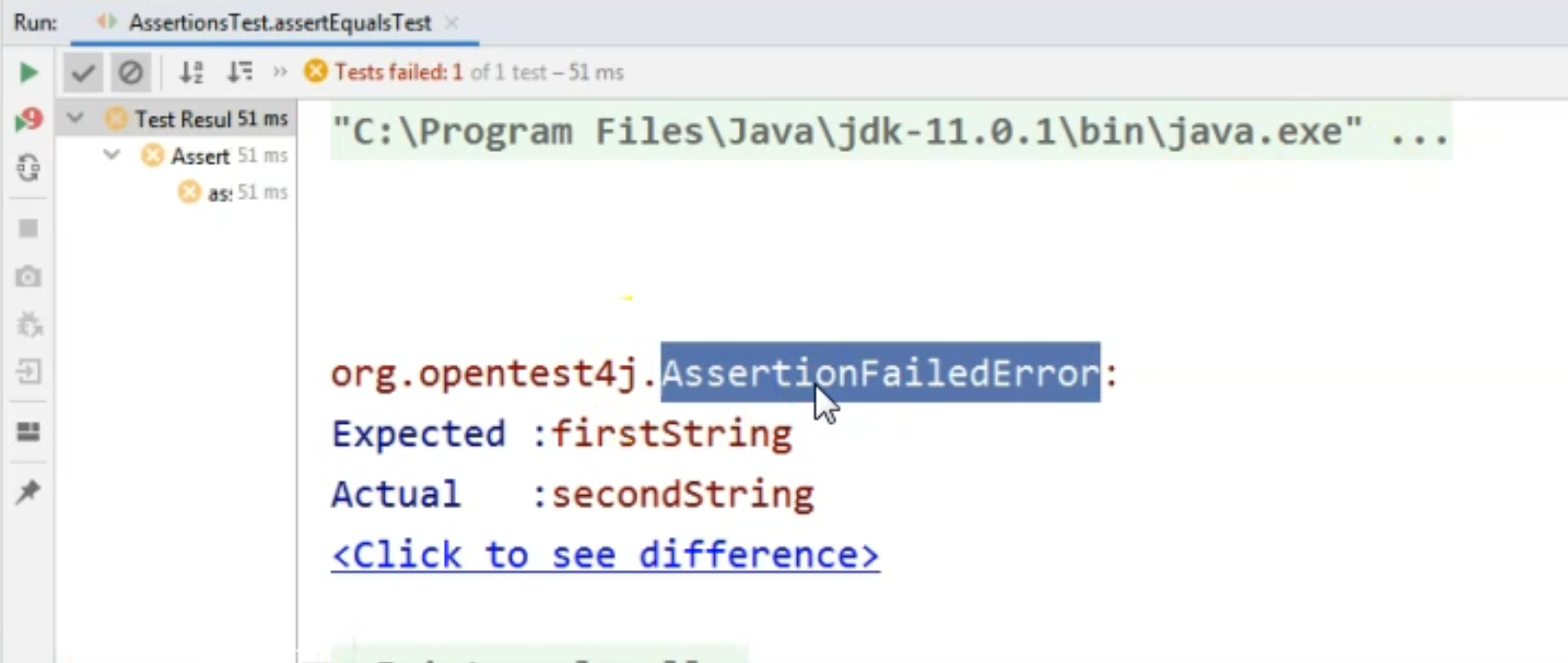If we run this test right now, we will see that we have a failure, but we don't have a specific message.

We only have the "AssertionFailedError" in the console, and we can see that the expected and the actual values were not the same.

Speaking about expected and actual values, if we're going back to the signature, we can see that the first parameter when we're calling the `assertEquals` needs to be the `expected` value.

The second parameter always needs to be the `actual` value.

We can also add a third parameter here, which would be a message to be displayed in the console in case we'll have a failure.

If we want to see something a bit more useful than the fact that we just had an "AssertionFailedError".

We could say something like, "The String values were not equal".

``````package junit5tests;

import org.junit.jupiter.api.Assertions;
import org.junit.jupiter.api.Test;

public class AssertionsTest {

@Test
void assertEqualsTest() {
assertEquals("firstString", "secondString", "The String " +
"values were not equal");
}
}
``````

Of course, this is obvious, but in the case of your tests, maybe your Assertions will require a message that is more meaningful to what you are testing.

If we run this test again, we will see that we now have the specified message in the console in addition to the test result.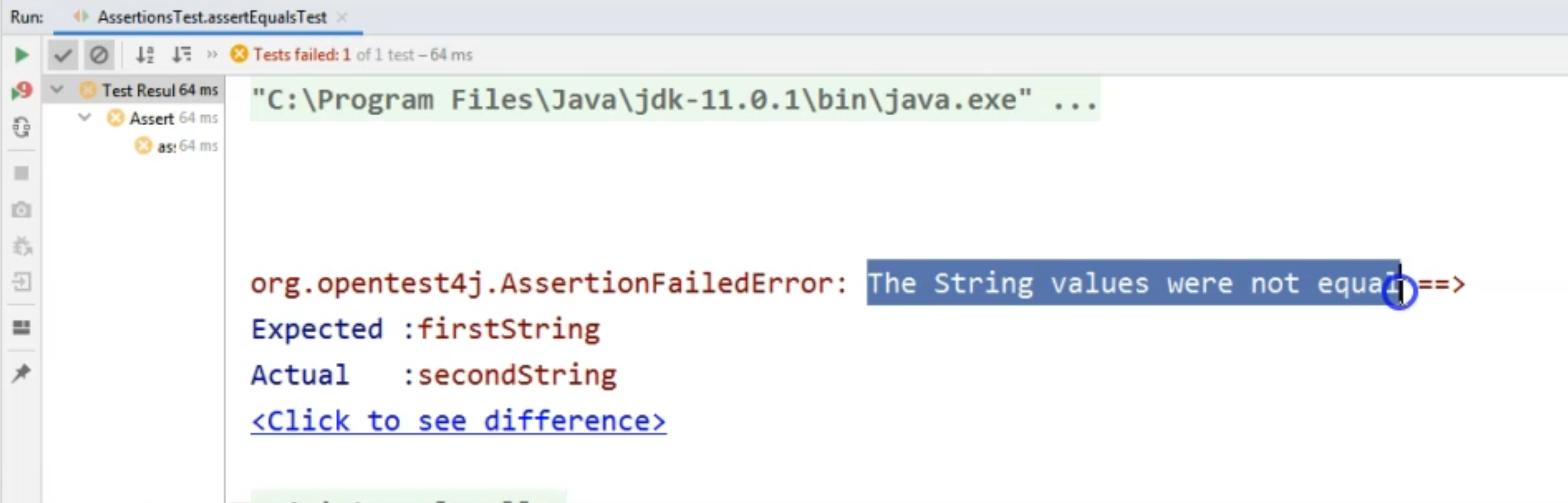The assertion failed error had a message saying that the "String values were not equal".

Again, we have the "Expected" and "Actual values" listed in the console.

As we've seen before, when we are using the `assertEquals`, we can compare different values, which are of a primitive type or a String, or even an object.

We can also compare lists of items.

Let's say we wanted to compare two lists of Strings to see that the values in both of the lists are exactly the same.

For that I will create a new test, and I will call it `assertEqualsListTest`.

Here, we will simply create a List of Strings and we will say `expectedValues =`, and this will just be `Arrays.asList()`.

We will just provide some values here - we will just say, `"firstString"`, `"secondString"`, and let's give it a `"thirdString"`.

Let's import the List.

Let's also create the list of actual values by just copy-pasting this one and renaming the list we pasted to `actualValues`.

In this case, we have two identical Lists of Strings.

We could also use the `Assertions.assertEquals()` method to compare the two lists.

We would say that we want to compare the `expectedValues` with the `actualValues`. \

Before we run this, we can simplify the code a little bit by using a static import for the `assertEquals` method. We can do that by hitting the "Alt+Enter" shortcut and choosing the "Add static import for 'org.junit.jupiter.api.Assertions.assertEquals" methods.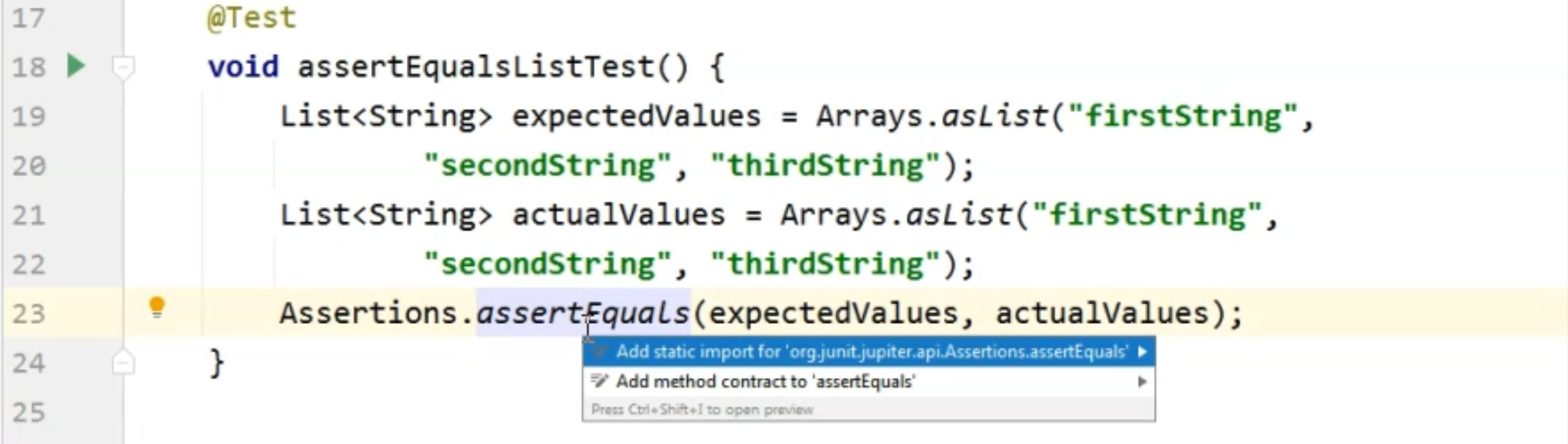In this case, the `assertEquals` has not been imported staticly in both of the tests that I have here.

``````package junit5tests;

import org.junit.jupiter.api.Assertions;
import org.junit.jupiter.api.Test;

import java.util.Arrays;
import java.util.List;

import static org.junit.jupiter.api.Assertions.*;

public class AssertionsTest {

@Test
void assertEqualsTest() {
assertEquals("firstString", "secondString", "The String " +
"values were not equal");
}

@Test
void assertEqualsListTest() {
List<String> expectedValues = Arrays.asList("firstString",
"secondString", "thirdString");
List<String> actualValues = Arrays.asList("firstString",
"secondString");
assertEquals(expectedValues, actualValues);
}
}
``````

Now we can just run this second test to see that it passes.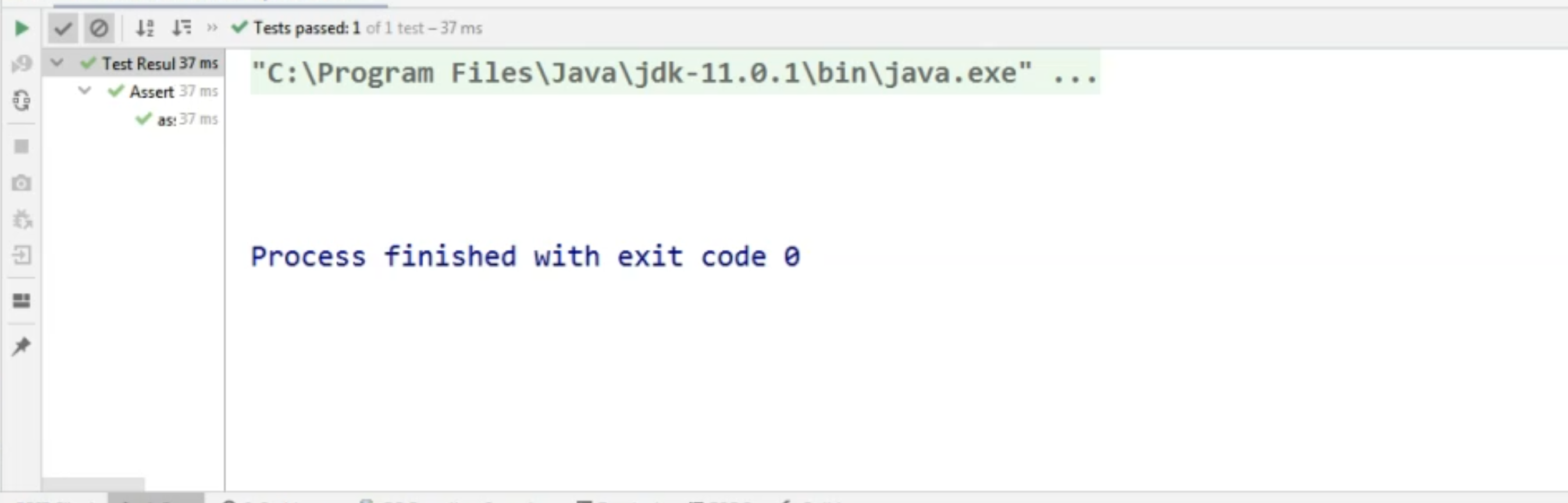It passed successfully.

Of course, we don't have any messages in the console because everything was okay.

However, if let's say we modify the list of `actualValues` and we simply remove the third item, if we rerun this test, we will now have an error, so there will be a failure.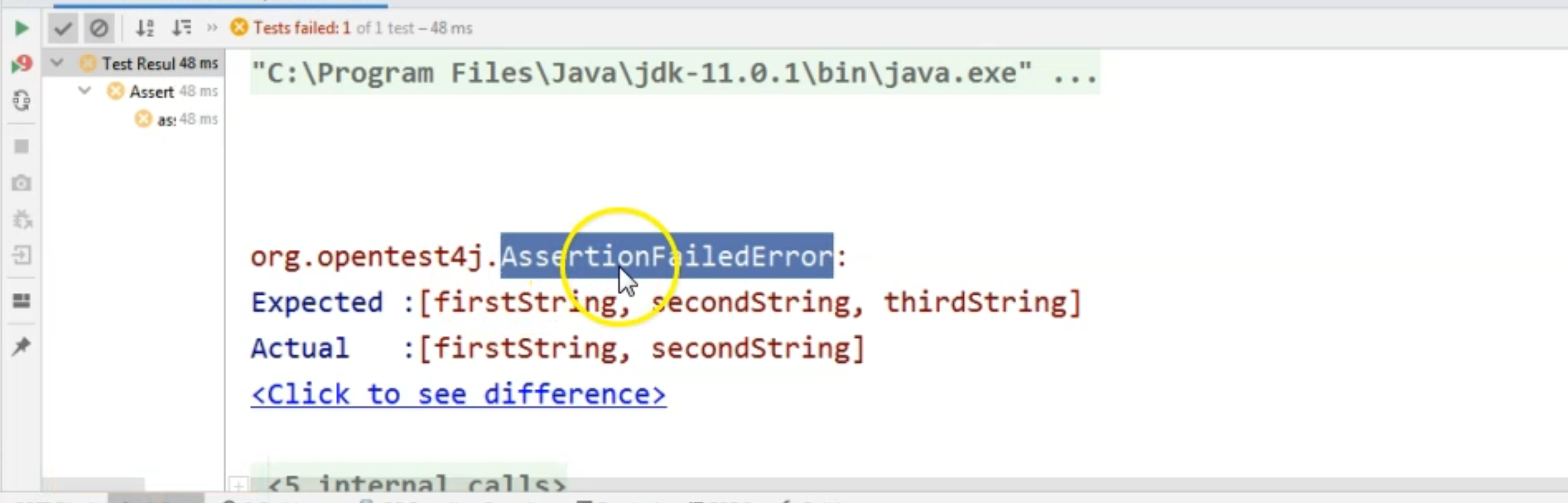Because we didn't provide any specific message, we will only see the AssertionFailedError in the console, and we will see that the Expected List of Strings has an extra item that the second list does not have.

In this case, the assertion failed because the list indeed did not have the exact same items.

Keep in mind that when used with Lists, the assertEquals will always check for the items belonging to the same position in both of the lists, meaning if the two lists have the same items, but they are not in the same order, then the Assertion will fail considering that the two lists are not identical.

If instead of Lists, we want to compare Arrays, we are going to use a different Assertion.

For that, we will use `assertArraysEqualsTest` Assertion, and I will create these brand new test methods for looking at this Assertion.

I will first create an array of `expectedValues`.

This will be simply the values `{1, 2, 3}`.

I will again, copy-paste this one, and I will create a new array `actualValues`.

To compare these two, now, I'm just going to say `assertArrayEquals` and I will just say `expectedValues` and `actualValues`.

Now, in my case, the two arrays are exactly the same, so they have the same values in the exact same order.

``````package junit5tests;

import org.junit.jupiter.api.Assertions;
import org.junit.jupiter.api.Test;

import java.util.Arrays;
import java.util.List;

import static org.junit.jupiter.api.Assertions.*;

public class AssertionsTest {

@Test
void assertEqualsTest() {
assertEquals("firstString", "secondString", "The String " +
"values were not equal");
}

@Test
void assertEqualsListTest() {
List<String> expectedValues = Arrays.asList("firstString",
"secondString", "thirdString");
List<String> actualValues = Arrays.asList("firstString",
"secondString");
assertEquals(expectedValues, actualValues);
}

@Test
void assertArraysEqualsTest() {
int[] expectedValues = {1, 5, 3};
int[] actualValues = {1, 2, 3};
assertArrayEquals(expectedValues, actualValues);
}
}
``````

I will run the test and the first time we are going to run the test, this will be successful, because as I said, the two arrays are exactly the same.

As you can see, all is green and there is no error.

However, if, let's say, we change the first array and we remove one of the items and rerun the test, we will now see an "AssertionFailedError" because the two arrays are different.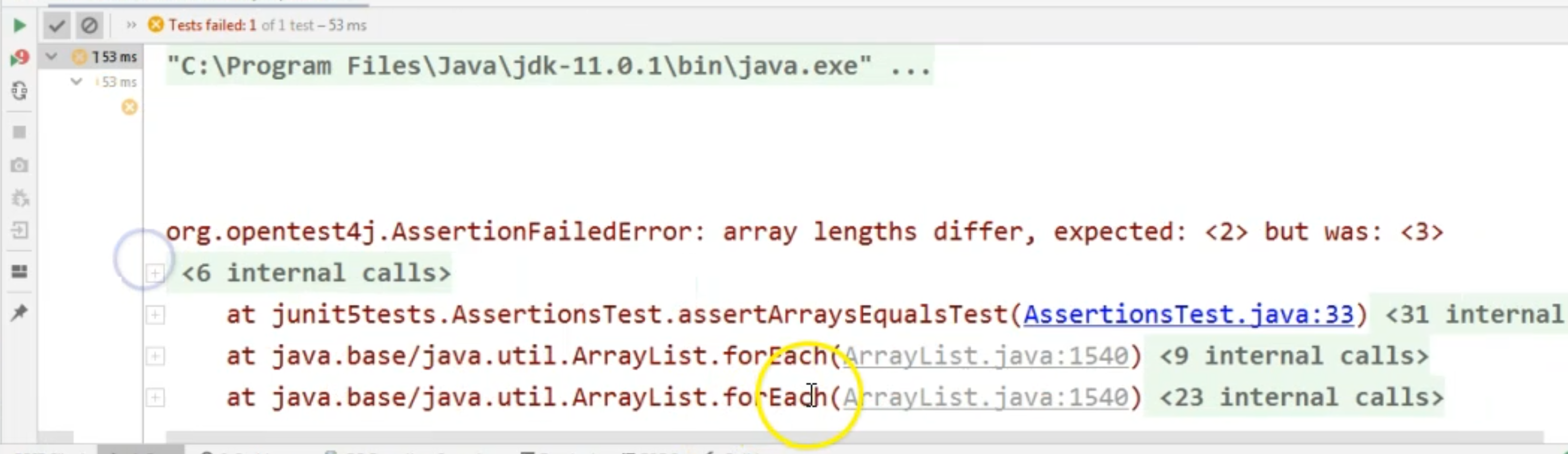As the Assertion message says, the length is different between the two anyway, so the lengths differ.

If, let's say, we try this again, but instead of `2`, we will say `5` on the second position.

Now we will rerun the test and they have the exact same length, but this time the assertion will fail because at index `1`, which means the second item in this array, the values don't match.

So the second item in the first array does not have the same value as the element in the second array.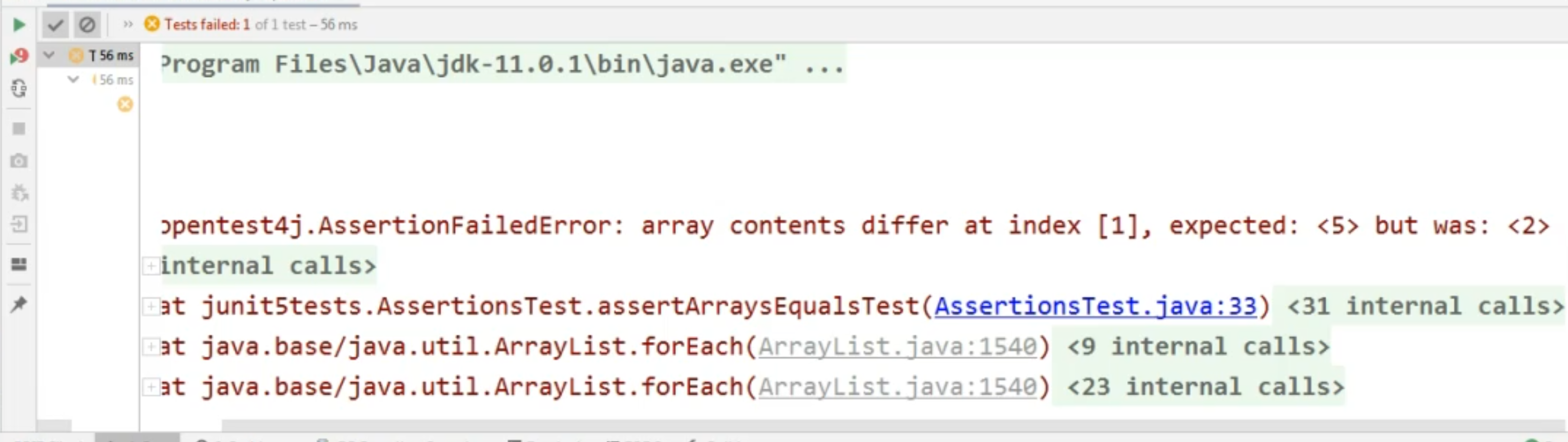In this case, the message is rather clear. We know exactly where the problem occurred.

Let's take a look at a third Assertion.

This one, again, is very popular and it's the `assertTrue` assertion.

Here, I'm just going to easily prove that this works and how this works by saying `assertTrue(false,)` - let's just also give it a message "This Boolean condition did not evaluate to true".

``````    @Test
void assertTrueTest() {
assertTrue(false, "This boolean condition did not evaluate to true");
}
``````

Of course, the parameter, when we're calling the Assertion, will be a boolean, so it will be something that evaluates to a boolean condition.

Whether it's an expression or a code to a different method, the result has to be a boolean when we are calling `assertTrue`.

Let's run this test and let's see that test indeed fails, and that we see the expected message in the console.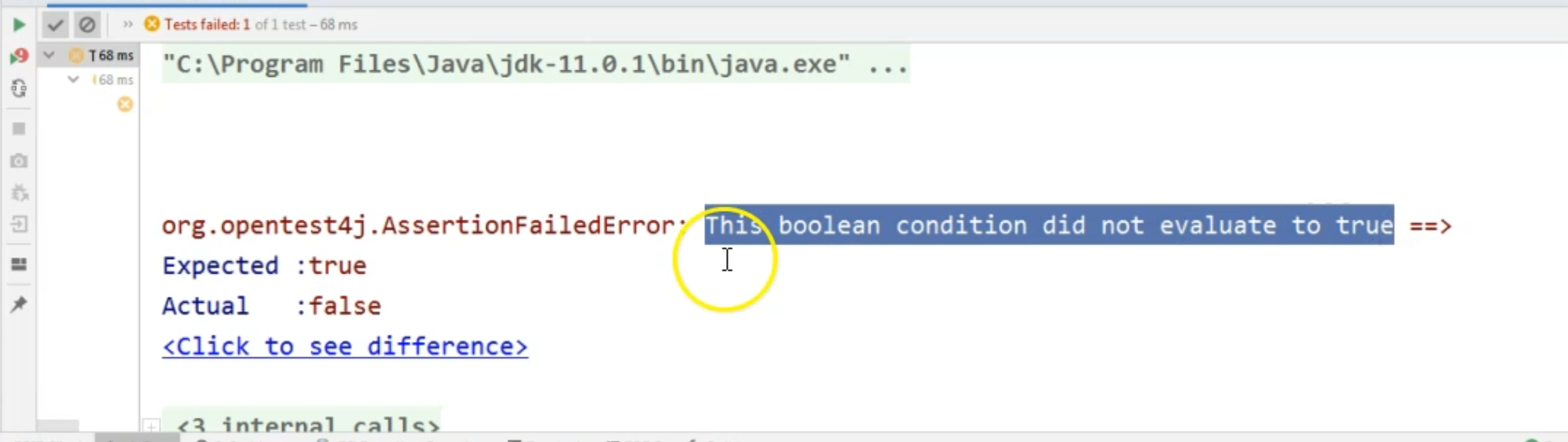As you can see, yes, "This boolean condition did not evaluate to true", is the message we will see along with what was the "Expected" value and what is the "Actual" value.

Now, similarly to `assertTrue`, there is also an assertion - namely, `assertFalse`.

Here we can say `assertFalse(false)`, and this assertion, exactly like `assertTrue`, requires a boolean condition to be passed to it, and the result of the assertion will depend on the value of that boolean.

``````    @Test
void assertTrueTest() {
assertFalse(false);
assertTrue(false, "This boolean condition did not evaluate to true");
}
``````

If we run the test again, the first assertion will pass, and the second one will be the one that fails.

Of course, similarly to `assertTrue` and `assertFalse`, we also have the `assertNotEquals` methods, in case we need to run these.

Just so you know, we have `assertEquals` and `assertNotEquals` available.

Let's take a look at another interesting assertion that we have - namely, the, `assertThrows` Assertion.

This one, as the name states, we'll just check that the code passed to it as a parameter, throws an exception that we expect to have.

They use it is as follows - `assertThrows()`, and here we need to provide what is the exception that we expect to see here.

For example, `NullPointerException.class`, and I will just pass a `null` value here.

``````    @Test
void assertThrowsTest() {
assertThrows(NullPointerException.class, null);
}
``````

You can imagine that instead of the `null`, you will actually have some code that executes.

For example, you might be calling the API with some values and the API will give you a response.

In case that response is an Exception, and the Exception matches the expected Exception, then this assertion will be successful.

In our case, when we run this, we will have a successful result, as you can see in the console because, indeed, we have a `NullPointerException` here.

The last thing we will look at here is, `assertAll` Assertion.

This is basically saying that even though you have several assertions in one test method, and let's say one of the first ones fail, you still want all of the assertions to be evaluated.

You want the entire test to run, no matter whether the Assertions failed and you want to collect the results at the end.

In order to do that, you would just say `assertAll`, and here you would just need to specify which are the assertions that you are trying to evaluate.

I will write them each on a new line, and we're going to take a few of these that we've already used.

I will just copy the first assertion that we had here, and I will just say that the first one will be the `assertEquals`, and then we will want a second one - here I'm just going to put in the, `assertThrows`, which we know is going to be successful.

This first one, of course, will not be successful.

The second one will be, and let's add a third and last one, and let's say we also want a failure because we want to see how this assertion works.

This is what the syntax looks like.

``````    @Test
void assertAllTest() {
assertAll(
() ->  assertEquals("firstString", "secondString", "The String " +
"values were not equal"),
() -> assertThrows(NullPointerException.class, null),
() -> assertTrue(false, "This boolean condition did not evaluate to true"));
}
``````

Here, we have an `assertAll`, and we have three assertions specified that we are going to check for, and we're going to check for all of them without failing the test at the first failure.

When we run this test, we will see two failures and one pass because the second assertion was correct.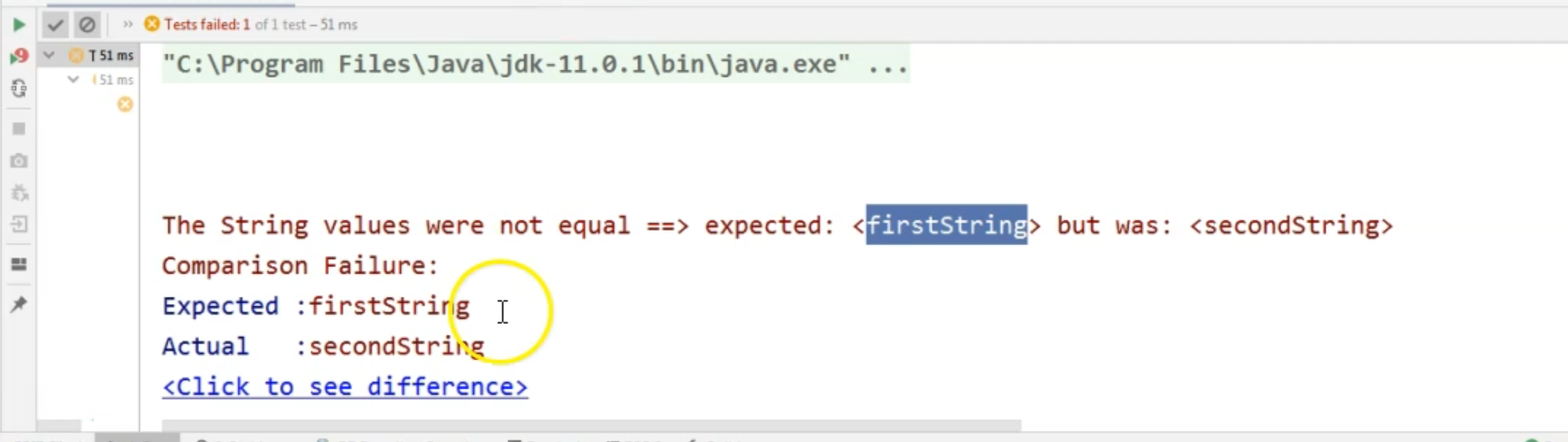If we look at the result, we can see that the Strings were not equal, so we are referring to the first assertion where we compared `firstString` with `secondString`.

Then we have a boolean condition that did not evaluate to true, which corresponds to the third assertion we had here.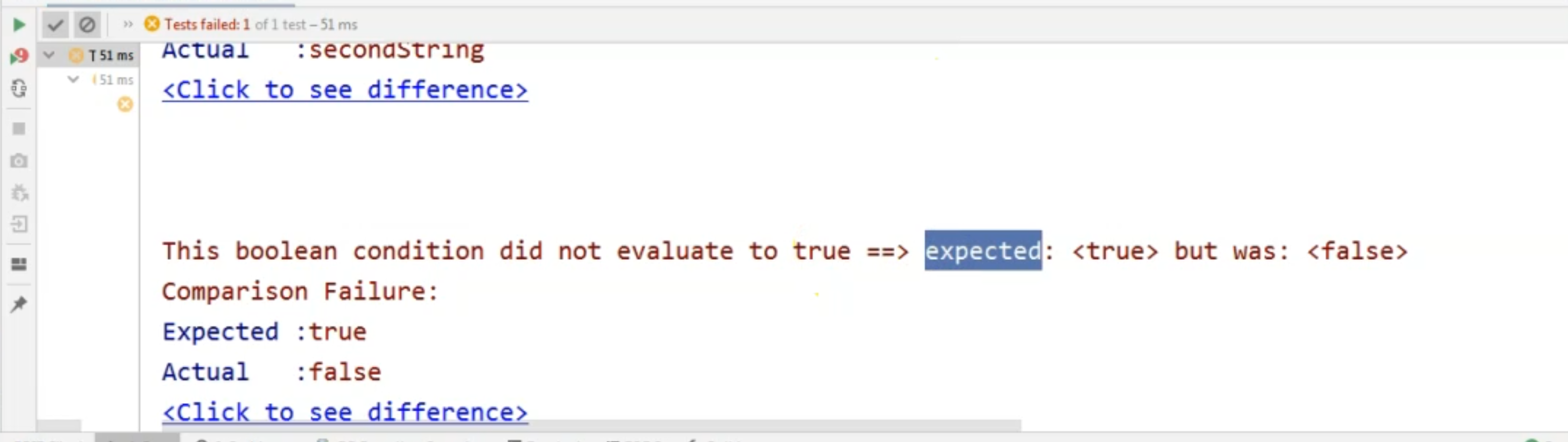Scrolling down a little bit, we will see that we did have multiple failures - namely, two failures.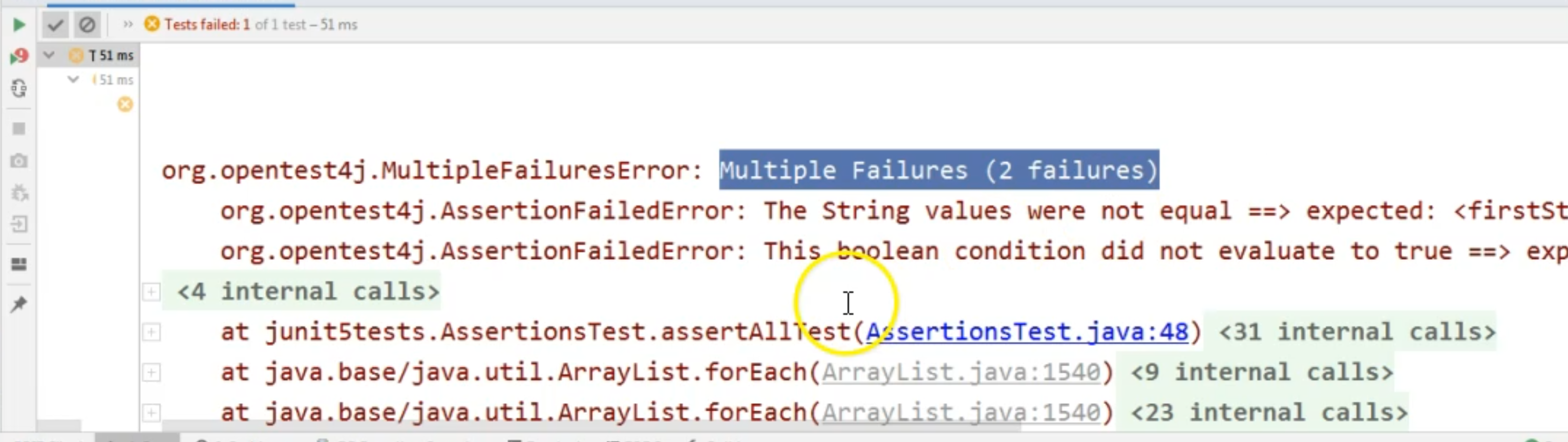These were due to the fact that the Strings were not equal in the first assertion, and that the boolean values also did not correspond to what we expected - the boolean value was not true.

This is it for the Assertions specific to JUnit 5 - which you can all find in the Assertions class from the JUnit Jupiter API package.